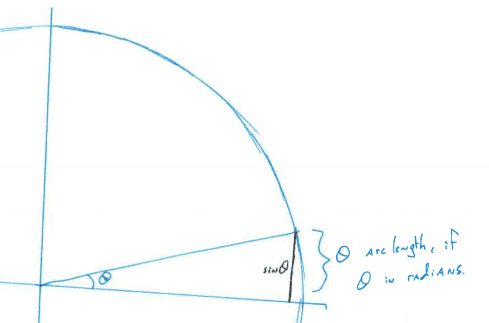A proof from the first sheet (SummerNT)

In class today, we were asked to explain what was wrong with the following proof:

Claim: As $x$ increases, the function

$displaystyle f(x)=frac{100x^2+x^2sin(1/x)+50000}{100x^2}$

approaches (gets arbitrarily close to) 1.

Proof: Look at values of $f(x)$ as $x$ gets larger and larger.

$f(5) approx 21.002$
$f(10)approx 6.0010$
$f(25)approx 1.8004$
$f(50)approx 1.2002$
$f(100) approx 1.0501$
$f(500) approx 1.0020$

These values are clearly getting closer to 1. QED

Of course, this is incorrect. Choosing a couple of numbers and thinking there might be a pattern does not constitute a proof.

But on a related note, these sorts of questions (where you observe a pattern and seek to prove it) can sometimes lead to strongly suspected conjectures, which may or may not be true. Here’s an interesting one (with a good picture over at SpikedMath):

Draw $2$ points on the circumference of a circle, and connect them with a line. How many regions is the circle divided into? (two). Draw another point, and connect it to the previous points with a line. How many regions are there now? Draw another point, connecting to the previous points with lines. How many regions now? Do this once more. Do you see the pattern? You might even begin to formulate a belief as to why it’s true.

But then draw one more point and its lines, and carefully count the number of regions formed in the circle. How many circles now? (It doesn’t fit the obvious pattern).

So we know that the presented proof is incorrect. But lets say we want to know if the statement is true. How can we prove it? Further, we want to prove it without calculus – we are interested in an elementary proof. How should we proceed?

Firstly, we should say something about radians. Recall that at an angle $theta$ (in radians) on the unit circle, the arc-length subtended by the angle $theta$ is exactly $theta$ (in fact, this is the defining attribute of radians). And the value $sin theta$ is exactly the height, or rather the $y$ value, of the part of the unit circle at angle $theta$. It’s annoying to phrase, so we look for clarification at the hastily drawn math below:The arc length subtended by theta has length theta. The value of sin theta is the length of the vertical line in black.

Note in particular that the arc length is longer than the value of $sin theta$, so that $sin theta < theta$. (This relies critically on the fact that the angle is positive). Further, we see that this is always true for small, positive $theta$. So it will be true that for large, positive $x$, we’ll have $sin frac{1}{x} < frac{1}{x}$. For those of you who know a bit more calculus, you might know that in fact, $sin(frac{1}{x}) = frac{1}{x} – frac{1}{x^33!} + O(frac{1}{t^5})$, which is a more precise statement.

What do we do with this? Well, I say that this allow us to finish the proof.

$dfrac{100x^2 + x^2 sin(1/x) + 50000}{100x^2} leq dfrac{100x^2 + x + 50000}{100x^2} = 1 + dfrac{1}{100x} + dfrac{50000}{100x^2}$

, and it is clear that the last two terms go to zero as $x$ increases. $spadesuit$

Finally, I’d like to remind you about the class webpage at the left – I’ll see you tomorrow in class.

This entry was posted in Brown University, Expository, Mathematics and tagged , , , , , , , . Bookmark the permalink.